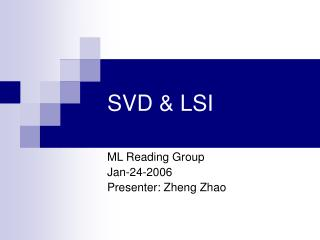DownloadDownload PresentationSVD & LSI

# SVD & LSI

Télécharger la présentation## SVD & LSI

- - - - - - - - - - - - - - - - - - - - - - - - - - - E N D - - - - - - - - - - - - - - - - - - - - - - - - - - -
##### Presentation Transcript

1. SVD & LSI ML Reading Group Jan-24-2006 Presenter: Zheng Zhao

2. SVD (Singular value decomposition) • Vector Norm • Matrix Norm • Singular value decomposition • The application of SVD

3. vector norm • A vector norm has the following properties. • 1. || x ||  0 (non-negative) • 2. || x || = 0 implies that all elements xi = 0 • 3. || x || =  || x || • 4. || x1 + x2 ||  || x1 || + || x2 || (triangular inequality) • Equivalence of norms

4. vector norm (cont.)

5. matrix (operator) norm A matrix (operator) norm has the following properties. 1. || A ||  0 (non-negative) 2. || A || = 0 implies that all elements xi = 0 3. || A || =  || A || 4. || A1 + A2 ||  || A1 || + || A2 || (triangular inequality) 5. || AB ||  || A || || B || (multiplicative property) An induced norm is defined as the following, for z = Ax measures how much A stretches x

6. matrix (operator) norm (cont.)

7. SVD • SVD- Singular value decomposition http://en.wikipedia.org/wiki/Singular_value_decomposition

8. Some Properties of SVD

9. Some Properties of SVD • That is, Ak is the optimal approximation in terms of the approximation error measured by the Frobenius norm, among all matrices of rank k • Forms the basics of LSI (Latent Semantic Indexing) in informational retrieval

10. Application of SVD • Pseudoinverse • Range, null space and rank • Matrix approximation • Other examples http://en.wikipedia.org/wiki/Singular_value_decomposition

11. LSI (Latent Semantic Indexing) • Problem Introduction • Latent Semantic Indexing • LSI • Query • Updating • An example • Some comments

12. Problem Introduction • Traditional term-matching method doesn’t work well in information retrieval • We want to capture the concepts instead of words. Concepts are reflected in the words. However, • One term may have multiple meaning • Different terms may have the same meaning.

13. LSI (Latent Semantic Indexing) • LSI approach tries to overcome the deficiencies of term-matching retrieval by treating the unreliability of observed term-document association data as a statistical problem. • The goal is to find effective models to represent the relationship between terms and documents. Hence a set of terms, which is by itself incomplete and unreliable, will be replaced by some set of entities which are more reliable indicants.

14. LSI, the Method • Document-Term M • Decompose M by SVD. • Approximating M using truncated SVD

15. LSI, the Method (cont.) Each row and column of A gets mapped into the k-dimensional LSI space, by the SVD.

16. Fundamental Comparison Quantities from the SVD Model • Comparing Two Terms: the dot product between two row vectors of reflects the extent to which two terms have a similar pattern of occurrence across the set of document. • Comparing Two Documents: dot product between two column vectors of • Comparing a Term and a Document

17. Query • A query q is also mapped into this space, by • Compare the similarity in the new space • Intuition: Dimension reduction through LSI brings together “related” axes in the vector space.

18. Updating • Recomposing • Expensive • Fold in Method New terms and documents have no effect on the representation of the preexisting terms and documents

19. Example

20. Example (cont.)

21. Example (cont. Mapping)

22. Example (cont. Query) Query: Application and Theory

23. Example (cont. Query)

24. Example (cont. fold in)

25. Example (cont. recomposing)

26. Choosing a value for k • LSI is useful only if k << n. • If k is too large, it doesn't capture the underlying latent semantic space; if k is too small, too much is lost. • No principled way of determining the best k; need to experiment.

27. How well does LSI work? • Effectiveness of LSI compared to regular term-matching depends on nature of documents. • Typical improvement: 0 to 30% better precision. • Advantage greater for texts in which synonymy and ambiguity are more prevalent. • Best when recall is high. • Costs of LSI might outweigh improvement. • SVD is computationally expensive; limited use for really large document collections • Inverted index not possible